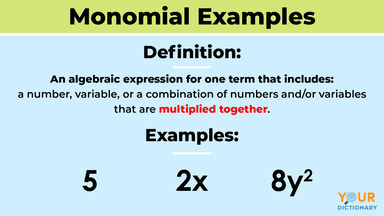# Examples of Monomials

A monomial is an expression in algebra that contains one term, like 3xy. Monomials include numbers, variables or multiple numbers and/or variables that are multiplied together. Any number all by itself is a monomial, like 5 or 2,700. A monomial can also be a variable, like "b" or "y." It can also be a combination of these, like 98b or xy. Explore the rules of monomials, monomial degrees and monomial examples.monomial examples

## Monomial Definition

One of the first math terms you learn in algebra is monomials. When it comes to understanding the definition of a monomial, it’s pretty easy. Mono means “one.” So, monomial functions are those expressions that only have the one term. While a monomial can be a single number, variable or combination of a number and variables, it can’t be a negative exponent. Therefore, monomials have two rules.

### The Rules of Monomials

Math always includes a few rules and monomials aren’t any different. There are two rules to remember about monomials. In these examples, the * symbol stands for multiplication.

1. A monomial multiplied by a monomial is also a monomial.

2 * 2 = 4 (a monomial)
2 * x = 2x
2 * 6 = 12
2 * y = 2y

2. A monomial multiplied by a constant (number) is also a monomial.

-13 * 7z = -91z (13 is the constant and 7z the monomial)
(⅛) * 8mn = -mn (⅛ is the constant and 8mn the monomial)
(⅕) * 5p = p (⅕ is the constant and 5p is the monomial)

## Examples: Numbers That Are Monomials

Now, it's time to really look at a few examples of monomials. Monomials are positive numbers. It doesn't matter how big they are, they are still a monomial. See a few examples of monomial numbers in action.

• 1, 2, 3, 4, 5, 6, 7, 8, 9, 10
• 100
• 500
• 1,000
• 5,000
• 10,000
• 3,598,772
• 4,000,000
• 14,000,000
• 14,100,300
• 20,000,000

## Examples: Variables That Are Monomials

It might be hard to think of a variable as a monomial, especially when you get to a group like abc. But remember one of the rules of monomials, a monomial multiplied by a monomial is still, you guessed it, a monomial. Therefore, variables multiplied by each other are also monomials. Look at a few examples.

• x
• y
• xy
• abc
• mx
• n
• b
• w
• l
• s
• bxy
• a
• ax

## Examples: Combinations of Numbers and Variables That Are Monomials

Numbers and variables aren't going to stand alone when it comes to monomials. They can work together. Therefore, 645a is still a monomial. Explore a few other examples of combinations of monomials.

• 1x
• 2y²
• 32x³y
• 653abc
• 2g7g9g

## Degrees of a Monomial Function

You might have noticed in the combinations that some monomials have an exponent. For example, 2y² has an exponent of 2. It also has a degree of 2. In a monomial, you can add the exponents of the variables together to find the degree of a monomial function. The exponent for a constant is always 0, and the exponent for a variable that doesn't have an exponent listed is always 1. For example:

5ab3c4
5, exponent = 0
a, exponent = 1
b, exponent = 3
c, exponent = 4
The total degree of this equation is 0 + 1 + 3 + 4 = 8